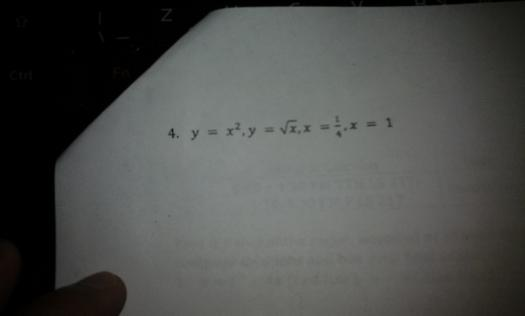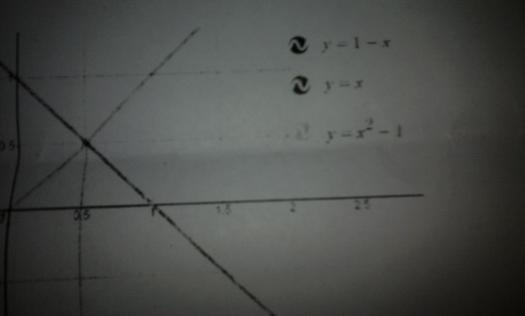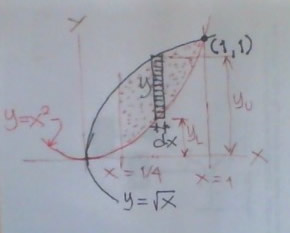# area of the region bounded

5 posts / 0 newarea of the region boundedJhun VertThe equation $y = x^2$ is an upward parabola and the $y = \sqrt{x}$ is a rightward parabola. The two has vertex at the origin and they meet at point (1, 1). The required area is dotted region in the figure below:The area of the rectangular element is y dx, and y is the difference between the top end and bottom end of the strip. In equation,
$dA = y \, dx = (y_U - y_L) \, dx$

You sum (integrate) it up and you're good to go.Thank you po..

• Mathematics inside the configured delimiters is rendered by MathJax. The default math delimiters are $$...$$ and $...$ for displayed mathematics, and $...$ and $...$ for in-line mathematics.